# Deep Spatio-Temporal Residual Networks for Citywide Crowd Flows Prediction

Junbo Zhang, Yu Zheng, Dekang Qi (Microsoft Research) 2017

Keras implementation : https://github.com/lucktroy/DeepST

## Introduction

• Forecating the flow of crowds
• In this paper, we predict two types fo crowd flows : inflow and outflow

• Inflow and outflow of crowds are affected by the following
• Spatial dependencies
• Temporal dependencies
• External influence : such as weather, events
• Contributions
• ST-ResNet employs convolution-based residual networks to model nearby and distance spatial dependencies between any two regions
• three categories of temporal properties : temporal closeness, period, and trend. ST-ResNet use three residual netowrks to model these, respectively
• ST-ResNet dynamically aggregates the output of the three aforementioned networks.

## Formulation of Crowd Flows Problem

• Region : we partition a city into an I*J grid map
• Inflow/outflow : Let P be a collection of trajectories at the tth time interval. For a grid (i, j) that lies at the ith row and jth column, the inflow and outflow of the crowds at the tiem interval t are defined respectively as $x_t^{in, i, j} = \sum_{T_r \in P} |{k > 1 |g_{k-1} \notin (i, j) \land g_k \in (i, j)}| \\ x_t^{out, i, j} = \sum_{T_r \in P} |{k \ge 1 |g_{k-1} \in (i, j) \land g_{k+1} \notin (i, j)}|$ where
• $T_r : g_1 \to g_2 \to ... \to g_{\left\vert T_r \right\vert}$ is a trajectory in P
• $g_k$ is the geospatial coordinate
• $g_k \in (i, j)$ means the point $g_k$ lies within grid (i, j), and vice versa
• $\left\vert \cdot \right\vert$ denotes the cardinality of a set

## Deep Spatio-Temporal Residual Networks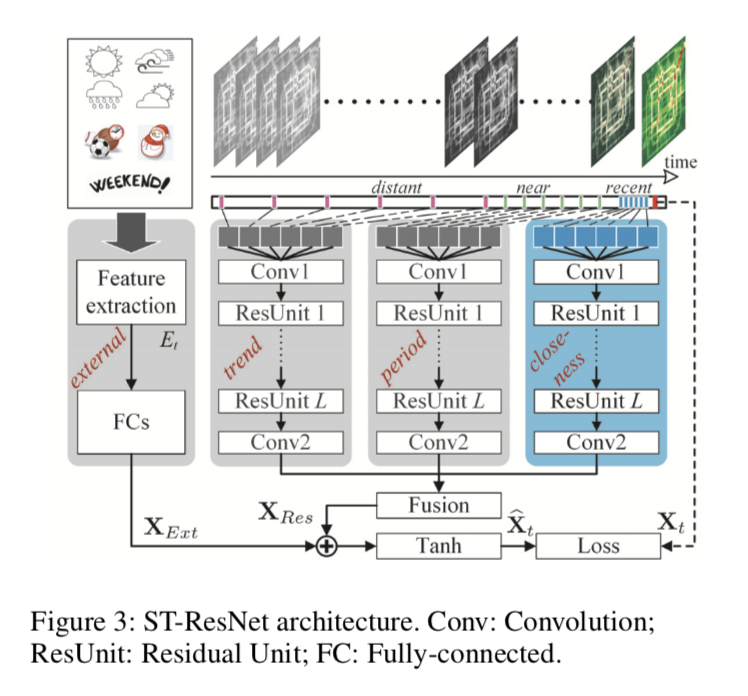• comprised of four major components modeling temporal closeness, period, trend, and external influence, respectively.

• First, we turn inflow and outflow throughout a city at each time interval into a 2-channel image-like matrix.

• Then, we divide the time axis into three fragments, denoting recent time, near history and distant history. The 2-channel flow matrics of intervals in each time fragment are the fed into the first three components seperately to model the aforementioned three temporal properties: closeness, period, and trend
• three components share the same network structure(Regisudal Unit sequence)
• The output of the three components are fused as $X_{Res}$ based on parameter metrics, which assign different weights to the results of different components in different regions.
• In the external component, we manually extract some feature form external datasets, such as weather conditions and events, feeding them into a two-layer fully-connected neural network.

• $X_{Res}$ and $X_{Ext}$ are integrated together. Then, the final output is mapped into [-1, 1] using Tanh function.

### Structures of the First Three Components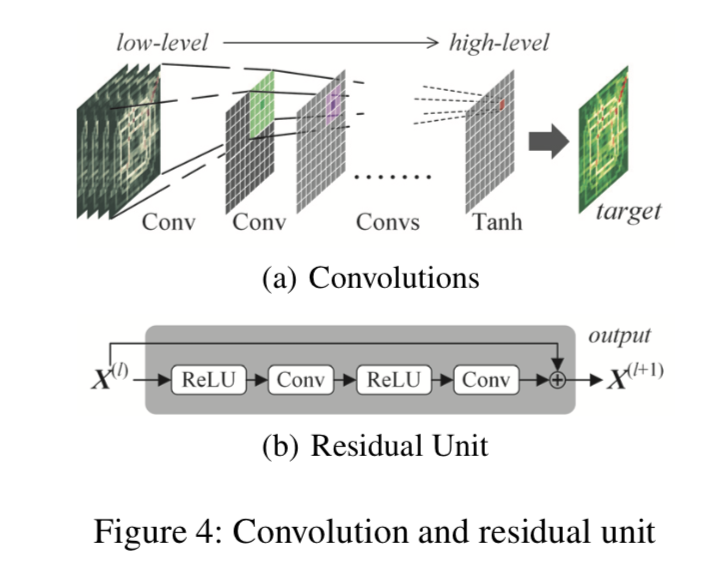• Do not user subsampling, but only convolutions
• closeness component
• $[X_{t-l_c}, X_{t-l_c-1}, ..., X_{t-1}]$ : concatnate them along with the first axis
• $X_c^{(0)} \in R^{2l_c \times I \times J}$ is followed by conv1
• Residual Unit : stack $L$ residual units to capture very large citywide dependencies
• Residual Unit combinations fo “ReLu + Convolution” and “BatchNormalization” is added before ReLu.
• On top of the $L^{th}$ residual unit, we append a convolutional layer conv2
• output of the closeness componet is $X_c^{(L+2)}$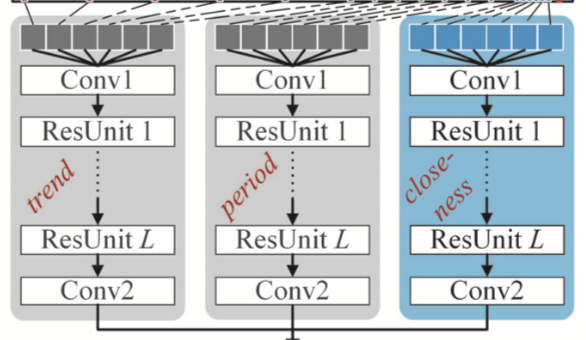• period component
• Assume that there are $l_p$ time intervals from the period fragment and the period is $p$ ;$[X_{t-l_p \cdot p}, X_{t-(l_p-1) \cdot p}, ..., X_{t-p}]$
• output : $X_p^{(L+2)}$
• in implementation, p is equal to one-day (daily periodicity)
• trend component
• $l_q$ is the length of the trend dependent sequence and q is the trend span
• input : $[X_{t-l_q \cdot q}, X_{t-(l_q-1) \cdot q}, ..., X_{t-q}]$
• output : $X_q^{(L+2)}$
• in implementation, q is equal to one-week(week trend)

### The Structure of the External Component

• mainly consider weather, holiday event, and metadata(DayOfWeek, Weekday/Weekend)

• stack two fully-connected layers upon $E_t$

• first layer : embedding layer
• second layer : to map low to high dimensions that have the same shape with $X_t$

### Fusion

• flows of two regions are all affected by closeness, period, and trend, but the degrees of influence may be very different ; parametric-matrix-based fusion
• $\circ$ is Hadamard product (i.e., element-wise multiplication)
• $W_c, W_p, W_q$ are learnable parameters

• fusing the external component
• objectives : minimizing mean squared error between the predicted flow matrix and the true flow matrix.

## Experiments

• Datasets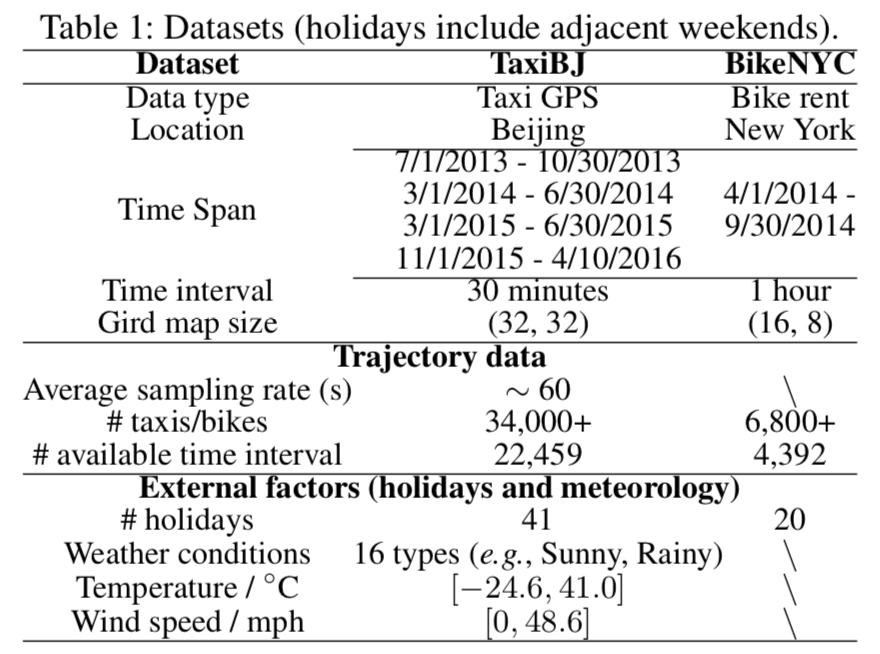• Baselines
• HA : historical data (previous week, same time)
• ARIMA, SARIMA, VAR
• ST-ANN : It first extracts spatial (nearby 8 regions’ values) and temporal (8 previous time intervals) features, then fed into an artificial neural network.
• DeepST : (Zhang et al. 2016)
• Preprocessing
• min-max normalization : [-1, 1] (tanh)
• one-hot encoding for external data
• Result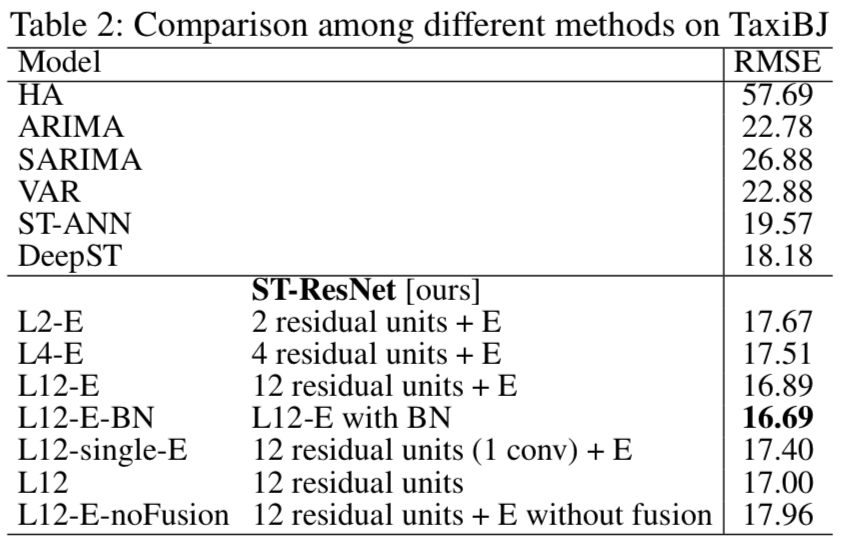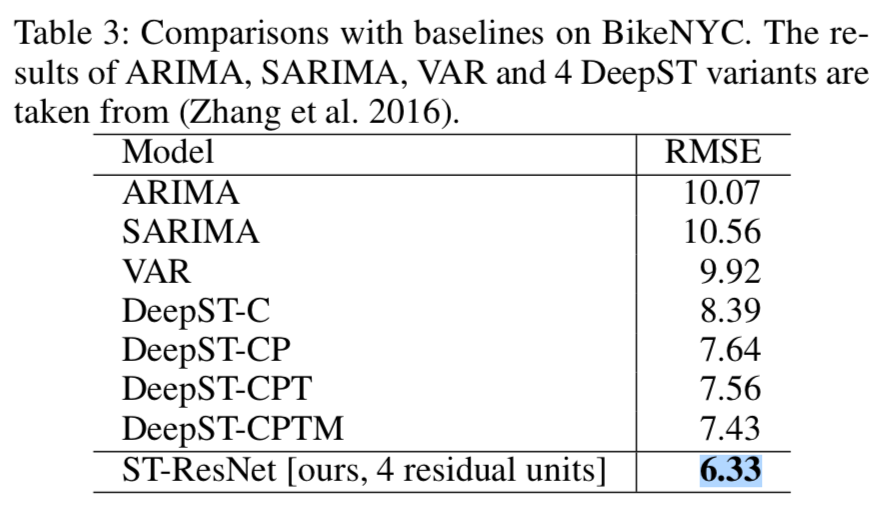Categories:

Updated: